• Matplotlib可能是python 2D-绘图领域使用最广泛的套件。它能让使用者很轻松的将数据图形化，并且提供多样化的输出格式。
• Matplotlib 教程 本文为译文，原文载于此，译文原载于此。本文欢迎转载，但请保留本段文字，尊重作者和译者的权益。谢谢。: ) 介绍 Matplotlib 可能是 Python 2D-绘图领域使用最广泛的套件。它能让使用者很...
 Matplotlib 教程

本文为译文，原文载于此，译文原载于此。本文欢迎转载，但请保留本段文字，尊重作者和译者的权益。谢谢。: )

介绍
Matplotlib 可能是 Python 2D-绘图领域使用最广泛的套件。它能让使用者很轻松地将数据图形化，并且提供多样化的输出格式。这里将会探索 matplotlib 的常见用法。

IPython 以及 pylab 模式
IPython 是 Python 的一个增强版本。它在下列方面有所增强：命名输入输出、使用系统命令（shell commands）、排错（debug）能力。我们在命令行终端给 IPython 加上参数 -pylab （0.12 以后的版本是 --pylab）之后，就可以像 Matlab 或者 Mathematica 那样以交互的方式绘图。
pylab
pylab 是 matplotlib 面向对象绘图库的一个接口。它的语法和 Matlab 十分相近。也就是说，它主要的绘图命令和 Matlab 对应的命令有相似的参数。
初级绘制
这一节中，我们将从简到繁：先尝试用默认配置在同一张图上绘制正弦和余弦函数图像，然后逐步美化它。
第一步，是取得正弦函数和预先函数的值：
from pylab import *

X = np.linspace(-np.pi, np.pi, 256,endpoint=True)
C,S = np.cos(X), np.sin(X)
X 是一个 numpy 数组，包含了从-pi到pi等间隔的 256 个值。C 和 S 则分别是这 256 个值对应的余弦和正弦函数值组成的numpy 数组。
你可以在 IPython 的交互模式下测试代码，也可以下载代码（下载链接就是这些示例图），然后执行：
python exercise_1.py
使用默认配置
Matplotlib 的默认配置都允许用户自定义。你可以调整大多数的默认配置：图片大小和分辨率（dpi）、线宽、颜色、风格、坐标轴、坐标轴以及网格的属性、文字与字体属性等。不过，matplotlib 的默认配置在大多数情况下已经做得足够好，你可能只在很少的情况下才会想更改这些默认配置。
from pylab import *

X = np.linspace(-np.pi, np.pi, 256,endpoint=True)
C,S = np.cos(X), np.sin(X)

plot(X,C)
plot(X,S)

show()默认配置的具体内容
下面的代码中，我们展现了 matplotlib 的默认配置并辅以注释说明，这部分配置包含了有关绘图样式的所有配置。代码中的配置与默认配置完全相同，你可以在交互模式中修改其中的值来观察效果。
# 导入 matplotlib 的所有内容（nympy 可以用 np 这个名字来使用）
from pylab import *

# 创建一个 8 * 6 点（point）的图，并设置分辨率为 80
figure(figsize=(8,6), dpi=80)

# 创建一个新的 1 * 1 的子图，接下来的图样绘制在其中的第 1 块（也是唯一的一块）
subplot(1,1,1)

X = np.linspace(-np.pi, np.pi, 256,endpoint=True)
C,S = np.cos(X), np.sin(X)

# 绘制余弦曲线，使用蓝色的、连续的、宽度为 1 （像素）的线条
plot(X, C, color="blue", linewidth=1.0, linestyle="-")

# 绘制正弦曲线，使用绿色的、连续的、宽度为 1 （像素）的线条
plot(X, S, color="green", linewidth=1.0, linestyle="-")

# 设置横轴的上下限
xlim(-4.0,4.0)

# 设置横轴记号
xticks(np.linspace(-4,4,9,endpoint=True))

# 设置纵轴的上下限
ylim(-1.0,1.0)

# 设置纵轴记号
yticks(np.linspace(-1,1,5,endpoint=True))

# 以分辨率 72 来保存图片
# savefig("exercice_2.png",dpi=72)

# 在屏幕上显示
show()改变线条的颜色和粗细
首先，我们以蓝色和红色分别表示余弦和正弦函数，而后将线条变粗一点。接下来，我们在水平方向拉伸一下整个图。
...
figure(figsize=(10,6), dpi=80)
plot(X, C, color="blue", linewidth=2.5, linestyle="-")
plot(X, S, color="red",  linewidth=2.5, linestyle="-")
...设置图片边界
当前的图片边界设置得不好，所以有些地方看得不是很清楚。
...
xlim(X.min()*1.1, X.max()*1.1)
ylim(C.min()*1.1, C.max()*1.1)
...
更好的方式是这样：
xmin ,xmax = X.min(), X.max()
ymin, ymax = Y.min(), Y.max()

dx = (xmax - xmin) * 0.2
dy = (ymax - ymin) * 0.2

xlim(xmin - dx, xmax + dx)
ylim(ymin - dy, ymax + dy)设置记号
我们讨论正弦和余弦函数的时候，通常希望知道函数在-pi和pi的值。这样看来，当前的设置就不那么理想了。
...
xticks( [-np.pi, -np.pi/2, 0, np.pi/2, np.pi])
yticks([-1, 0, +1])
...设置记号的标签
记号现在没问题了，不过标签却不大符合期望。我们可以把3.14当做是pi，但毕竟不够精确。当我们设置记号的时候，我们可以同时设置记号的标签。注意这里使用了 LaTeX。
...
xticks([-np.pi, -np.pi/2, 0, np.pi/2, np.pi],
[r'$-\pi$', r'$-\pi/2$', r'$0$', r'$+\pi/2$', r'$+\pi$'])

yticks([-1, 0, +1],
[r'$-1$', r'$0$', r'$+1$'])
...移动脊柱
坐标轴线和上面的记号连在一起就形成了脊柱（Spines，一条线段上有一系列的凸起，是不是很像脊柱骨啊~），它记录了数据区域的范围。它们可以放在任意位置，不过至今为止，我们都把它放在图的四边。
实际上每幅图有四条脊柱（上下左右），为了将脊柱放在图的中间，我们必须将其中的两条（上和右）设置为无色，然后调整剩下的两条到合适的位置——数据空间的 0 点。
...
ax = gca()
ax.spines['right'].set_color('none')
ax.spines['top'].set_color('none')
ax.xaxis.set_ticks_position('bottom')
ax.spines['bottom'].set_position(('data',0))
ax.yaxis.set_ticks_position('left')
ax.spines['left'].set_position(('data',0))
...添加图例
我们在图的左上角添加一个图例。为此，我们只需要在 plot 函数里以「键 - 值」的形式增加一个参数。
...
plot(X, C, color="blue", linewidth=2.5, linestyle="-", label="cosine")
plot(X, S, color="red",  linewidth=2.5, linestyle="-", label="sine")

legend(loc='upper left')
...给一些特殊点做注释
我们希望在关注的位置给两条函数曲线加上一个注释。首先，我们在对应的函数图像位置上画一个点；然后，向横轴引一条垂线，以虚线标记；最后，写上标签。
...

t = 2*np.pi/3
plot([t,t],[0,np.cos(t)], color ='blue', linewidth=2.5, linestyle="--")
scatter([t,],[np.cos(t),], 50, color ='blue')

annotate(r'$\sin(\frac{2\pi}{3})=\frac{\sqrt{3}}{2}$',
xy=(t, np.sin(t)), xycoords='data',
xytext=(+10, +30), textcoords='offset points', fontsize=16,

plot([t,t],[0,np.sin(t)], color ='red', linewidth=2.5, linestyle="--")
scatter([t,],[np.sin(t),], 50, color ='red')

annotate(r'$\cos(\frac{2\pi}{3})=-\frac{1}{2}$',
xy=(t, np.cos(t)), xycoords='data',
xytext=(-90, -50), textcoords='offset points', fontsize=16,
...精益求精
坐标轴上的记号标签被曲线挡住了，作为强迫症患者（雾）这是不能忍的。我们可以把它们放大，然后添加一个白色的半透明底色。这样可以保证标签和曲线同时可见。
...
for label in ax.get_xticklabels() + ax.get_yticklabels():
label.set_fontsize(16)
label.set_bbox(dict(facecolor='white', edgecolor='None', alpha=0.65 ))
...图像、子图、坐标轴和记号
到目前为止，我们都用隐式的方法来绘制图像和坐标轴。快速绘图中，这是很方便的。我们也可以显式地控制图像、子图、坐标轴。Matplotlib 中的「图像」指的是用户界面看到的整个窗口内容。在图像里面有所谓「子图」。子图的位置是由坐标网格确定的，而「坐标轴」却不受此限制，可以放在图像的任意位置。我们已经隐式地使用过图像和子图：当我们调用 plot 函数的时候，matplotlib 调用 gca() 函数以及 gcf() 函数来获取当前的坐标轴和图像；如果无法获取图像，则会调用 figure() 函数来创建一个——严格地说，是用 subplot(1,1,1) 创建一个只有一个子图的图像。
图像
所谓「图像」就是 GUI 里以「Figure #」为标题的那些窗口。图像编号从 1 开始，与 MATLAB 的风格一致，而于 Python 从 0 开始编号的风格不同。以下参数是图像的属性：
参数 默认值 描述 num 1 图像的数量 figsize figure.figsize 图像的长和宽（英寸） dpi figure.dpi 分辨率（点/英寸） facecolor figure.facecolor 绘图区域的背景颜色 edgecolor figure.edgecolor 绘图区域边缘的颜色 frameon True 是否绘制图像边缘
这些默认值可以在源文件中指明。不过除了图像数量这个参数，其余的参数都很少修改。
你在图形界面中可以按下右上角的 X 来关闭窗口（OS X 系统是左上角）。Matplotlib 也提供了名为 close 的函数来关闭这个窗口。close 函数的具体行为取决于你提供的参数：
不传递参数：关闭当前窗口；传递窗口编号或窗口实例（instance）作为参数：关闭指定的窗口；all：关闭所有窗口。
和其他对象一样，你可以使用 setp 或者是 set_something 这样的方法来设置图像的属性。
子图
你可以用子图来将图样（plot）放在均匀的坐标网格中。用 subplot 函数的时候，你需要指明网格的行列数量，以及你希望将图样放在哪一个网格区域中。此外，gridspec 的功能更强大，你也可以选择它来实现这个功能。坐标轴
坐标轴和子图功能类似，不过它可以放在图像的任意位置。因此，如果你希望在一副图中绘制一个小图，就可以用这个功能。记号
良好的记号是图像的重要组成部分。Matplotlib 里的记号系统里的各个细节都是可以由用户个性化配置的。你可以用 Tick Locators 来指定在那些位置放置记号，用 Tick Formatters 来调整记号的样式。主要和次要的记号可以以不同的方式呈现。默认情况下，每一个次要的记号都是隐藏的，也就是说，默认情况下的次要记号列表是空的——NullLocator。
Tick Locators
下面有为不同需求设计的一些 Locators。
类型 说明 NullLocator No ticks.IndexLocator Place a tick on every multiple of some base number of points plotted.FixedLocator Tick locations are fixed.LinearLocator Determine the tick locations.MultipleLocator Set a tick on every integer that is multiple of some base.AutoLocator Select no more than n intervals at nice locations.LogLocator Determine the tick locations for log axes.这些 Locators 都是 matplotlib.ticker.Locator 的子类，你可以据此定义自己的 Locator。以日期为 ticks 特别复杂，因此 Matplotlib 提供了 matplotlib.dates 来实现这一功能。
其他类型的图
接下来的内容是练习。请运用你学到的知识，从提供的代码开始，实现配图所示的效果。具体的答案可以点击配图下载。
普通图
from pylab import *

n = 256
X = np.linspace(-np.pi,np.pi,n,endpoint=True)
Y = np.sin(2*X)

plot (X, Y+1, color='blue', alpha=1.00)
plot (X, Y-1, color='blue', alpha=1.00)
show()散点图
from pylab import *

n = 1024
X = np.random.normal(0,1,n)
Y = np.random.normal(0,1,n)

scatter(X,Y)
show()条形图
from pylab import *

n = 12
X = np.arange(n)
Y1 = (1-X/float(n)) * np.random.uniform(0.5,1.0,n)
Y2 = (1-X/float(n)) * np.random.uniform(0.5,1.0,n)

bar(X, +Y1, facecolor='#9999ff', edgecolor='white')
bar(X, -Y2, facecolor='#ff9999', edgecolor='white')

for x,y in zip(X,Y1):
text(x+0.4, y+0.05, '%.2f' % y, ha='center', va= 'bottom')

ylim(-1.25,+1.25)
show()等高线图
from pylab import *

def f(x,y): return (1-x/2+x**5+y**3)*np.exp(-x**2-y**2)

n = 256
x = np.linspace(-3,3,n)
y = np.linspace(-3,3,n)
X,Y = np.meshgrid(x,y)

contourf(X, Y, f(X,Y), 8, alpha=.75, cmap='jet')
C = contour(X, Y, f(X,Y), 8, colors='black', linewidth=.5)
show()灰度图（Imshow）

from pylab import *

def f(x,y): return (1-x/2+x**5+y**3)*np.exp(-x**2-y**2)

n = 10
x = np.linspace(-3,3,4*n)
y = np.linspace(-3,3,3*n)
X,Y = np.meshgrid(x,y)
imshow(f(X,Y)), show()饼状图
from pylab import *

n = 20
Z = np.random.uniform(0,1,n)
pie(Z), show()量场图（Quiver Plots）
from pylab import *

n = 8
X,Y = np.mgrid[0:n,0:n]
quiver(X,Y), show()网格
from pylab import *

axes = gca()
axes.set_xlim(0,4)
axes.set_ylim(0,3)
axes.set_xticklabels([])
axes.set_yticklabels([])

show()多重网格
from pylab import *

subplot(2,2,1)
subplot(2,2,3)
subplot(2,2,4)

show()极轴图
from pylab import *

axes([0,0,1,1])

N = 20
theta = np.arange(0.0, 2*np.pi, 2*np.pi/N)
width = np.pi/4*np.random.rand(N)
bars = bar(theta, radii, width=width, bottom=0.0)

bar.set_facecolor( cm.jet(r/10.))
bar.set_alpha(0.5)

show()3D 图
from pylab import *
from mpl_toolkits.mplot3d import Axes3D

fig = figure()
ax = Axes3D(fig)
X = np.arange(-4, 4, 0.25)
Y = np.arange(-4, 4, 0.25)
X, Y = np.meshgrid(X, Y)
R = np.sqrt(X**2 + Y**2)
Z = np.sin(R)

ax.plot_surface(X, Y, Z, rstride=1, cstride=1, cmap='hot')

show()手稿结束篇
Matplotlib 能有今天这样强大的功能和广泛的使用得益于大量的文档和社区开发者。这里提供一些有益的链接。
入门教程
Pyplot tutorialImage tutorialText tutorialArtist tutorialPath tutorialTransforms tutorial
Matplotlib 文档
用户指南常见问题及回答截屏
随代码分发的文档
Matplotlib 的代码是自文档（Self-documented）的。你可以在 Python 中快速查询某个命令的用法。

>>> from pylab import *
>>> help(plot)
Help on function plot in module matplotlib.pyplot:

plot(*args, **kwargs)
Plot lines and/or markers to the
:class:~matplotlib.axes.Axes.  *args* is a variable length
argument, allowing for multiple *x*, *y* pairs with an
optional format string.  For example, each of the following is
legal::

plot(x, y)         # plot x and y using default line style and color
plot(x, y, 'bo')   # plot x and y using blue circle markers
plot(y)            # plot y using x as index array 0..N-1
plot(y, 'r+')      # ditto, but with red plusses

If *x* and/or *y* is 2-dimensional, then the corresponding columns
will be plotted.
...
画廊
Matplotlib 画廊 也非常有用。其中的例子都有配图和对应的代码，当你不知道某一个效果如何实现的时候，你可以在这里找找。
哦，这里还有一个小一点的画廊。
邮件列表
你可以在用户邮件列表提问，或者在开发者邮件列表里交流技术。

译注：邮件列表是一个很正式的交流平台，其内的每一封邮件，列表的收听者都会看到，所以请不要在邮件列表灌水或发表质量低劣的文章。

快速查询
你可以在这里找到 Matplotlib 主要的属性表，以便按照需求个性化配置你的输出图样。
中文乱码解决方法
注意绘图时中文的解决方法, 在.py文件头部加上如下内容：
# -*- coding: utf-8 -*-
from pylab import *
mpl.rcParams['font.sans-serif'] = ['SimHei'] #指定默认字体 4
mpl.rcParams['axes.unicode_minus'] = False #解决保存图像是负号'-'显示为方块的问题 
可以根据所画图形的需要 在下面的链接里选择相应的图形 进行修改，绘出自己所需的图像。
example： http://matplotlib.org/examples/index.html, gallery：http://matplotlib.org/gallery.html
相关资源链接：
Matplotlib Tutorial 中文翻译的 http://reverland.org/python/2012/09/07/matplotlib-tutorial/ 。
用Python做科学计算 http://sebug.net/paper/books/scipydoc/inde
参考文献：  1、https://blog.csdn.net/u011497262/article/details/52325705 2018.5.24  2、https://liam0205.me/2014/09/11/matplotlib-tutorial-zh-cn/ 2018.5.24  3、https://www.cnblogs.com/laoniubile/p/5893286.html 2018.5.24  4、http://www.cnblogs.com/laoniubile/p/5891788.html 2018.5.24  5、http://reverland.org/python/2012/09/07/matplotlib-tutorial/ 2018.5.24  6、https://www.cnblogs.com/nju2014/p/5707980.html 2018.5.24  7、https://matplotlib.org/examples/index.html 2018.5.24  8、https://matplotlib.org/gallery.html 2018.5.24  9、https://matplotlib.org/tutorials/index.html 2018.5.24  10、http://www.labri.fr/perso/nrougier/teaching/matplotlib/ 2018.5.24（原版文档）
展开全文• Matplotlib 入门教程， Introduction to Matplotlib and basic line 英文地址https://pythonprogramming.net/matplotlib-intro-tutorial/
• matplotlib简介 如果你在大学里参加过数学建模竞赛或者是用过MATLAB的话，相比会对这一款软件中的画图功能印象深刻。MATLAB可以做出各种函数以及数值分布图像非常的好用和方便。如果你没用过呢也没关系，知道这么回...
• Matplotlib 数据可视化模块的使用教程Matplotlib 能够创建多数类型的图表， 如条形图， 散点图， 条形图， 饼图， 堆叠图， 3D 图和地图图表等
• 无涯教程网(learnfk)整理提供:Matplotlib是用于数据可视化的最受欢迎的Python软件包之一
• Matplotlib教程 本文为译文，原文载于此，译文原载于此。本文欢迎转载，但请保留本段文字，尊重作者和译者的权益。谢谢。: ) 本文是在原文和译文的基础上，稍作调整，调整为基于Jupyter Notebook 的代码。如想...
Matplotlib教程

本文为译文，原文载于此，译文原载于此。本文欢迎转载，但请保留本段文字，尊重作者和译者的权益。谢谢。: )

本文是在原文和译文的基础上，稍作调整，调整为基于Jupyter Notebook 的代码。如想观看原文和译文，请戳上面两个链接。 介绍 Matplotlib可能是Python 2D-绘图领域使用最广泛的包。它能让使用者很轻松的将数据图形化，并且提供多样化的输出格式，这里将会探索Matplotlib的常见用法。 初级绘制 这一节中，首先导入我们需要的包，从简到繁：先尝试用默认配置在同一张图上绘制正弦和余弦函数的图像，然后逐步美化它。（代码基于Jupyter Notebook） 第一步，导入包，取得正弦函数和余弦函数的值：
import numpy as np
import pandas as pd
import matplotlib.pyplot as plt

X = np.linspace(-np.pi,np.pi,256,endpoint=True)
C,S =np.cos(X),np.sin(X)

X 是一个 numpy 数组，包含了从 −π 到 +π 等间隔的 256 个值。C 和 S 则分别是这 256 个值对应的余弦和正弦函数值组成的 numpy 数组。 使用默认配置
plt.plot(X,C)
plt.plot(X,S)
plt.show()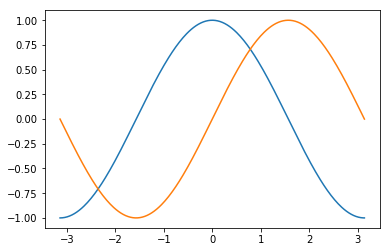默认配置的具体内容 Matplotlib 的默认配置都允许用户自定义。你可以调整大多数的默认配置：图片大小和分辨率（dpi）、线宽、颜色、风格、坐标轴、坐标轴以及网格的属性、文字与字体属性等。不过，Matplotlib 的默认配置在大多数情况下已经做得足够好，你可能只在很少的情况下才会想更改这些默认配置。下面的代码中，我们展现了 Matplotlib 的默认配置并辅以注释说明，这部分配置包含了有关绘图样式的所有配置。
# 创建一个 8 * 6 点（point）的图，并设置分辨率为 80
plt.figure(figsize=(8,6), dpi=80)

# 创建一个新的 1 * 1 的子图，接下来的图样绘制在其中的第 1 块（也是唯一的一块）
plt.subplot(1,1,1)

#从 *−π* 到 *+π* 等间隔的 256 个值，且包含端点值。
X = np.linspace(-np.pi, np.pi, 256,endpoint=True)
C,S = np.cos(X), np.sin(X)

# 绘制余弦曲线，使用蓝色的、连续的、宽度为 1.5 （像素）的线条
plt.plot(X, C, color="blue", linewidth=1.5, linestyle="-")

# 绘制正弦曲线，使用红色的、连续的、宽度为 1.5 （像素）的线条
plt.plot(X, S, color="red", linewidth=1.5, linestyle="-")

# 设置横轴的上下限
plt.xlim(-3.3,3.3)

# 设置横轴记号
plt.xticks(np.linspace(-3,3,7,endpoint=True))

# 设置纵轴的上下限
plt.ylim(-1.1,1.1)

# 设置纵轴记号
plt.yticks(np.linspace(-1,1,9,endpoint=True))

# 将图片保存为png格式,分辨率 100 ,如果是空白图片，你可以查阅相关知识
plt.savefig('exercice_2.png',dpi=100)

# 在屏幕上显示
plt.show()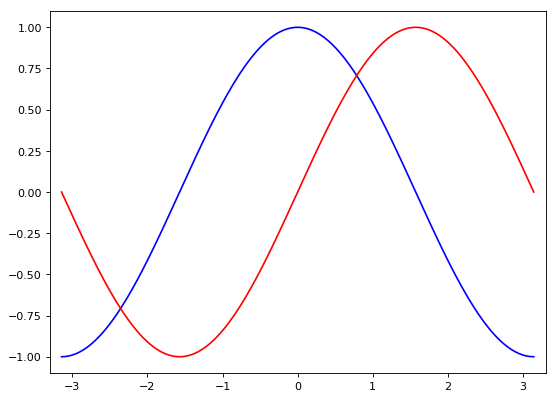改变线条的颜色和粗细 首先，我们以蓝色和红色分别表示余弦和正弦函数，而后将线条变粗一点。接下来，我们在水平方向拉伸一下整个图。
plt.plot(X, C, color="blue", linewidth=2.5, linestyle="-")
plt.plot(X, S, color="red",  linewidth=2.5, linestyle="-")

设置图片边界 当前的图片边界设置得不好，所以有些地方看得不是很清楚。
xmin ,xmax = X.min(), X.max()
ymin, ymax = C.min(), C.max()
dx = (xmax - xmin) * 0.2
dy = (ymax - ymin) * 0.2
plt.xlim(xmin - dx, xmax + dx)
plt.ylim(ymin - dy, ymax + dy)

设置记号和标签 我们讨论正弦和余弦函数的时候，通常希望知道函数在 ±π 和 ±π/2 的值。当我们设置记号的时候，我们可以同时设置记号的标签。注意这里使用了LaTeX。
plt.xticks([-np.pi, -np.pi/2, 0, np.pi/2, np.pi],
[r'$-\pi$', r'$-\pi/2$', r'$0$', r'$+\pi/2$', r'$+\pi$'])

plt.yticks([-1, 0, +1],
[r'$-1$', r'$0$', r'$+1$'])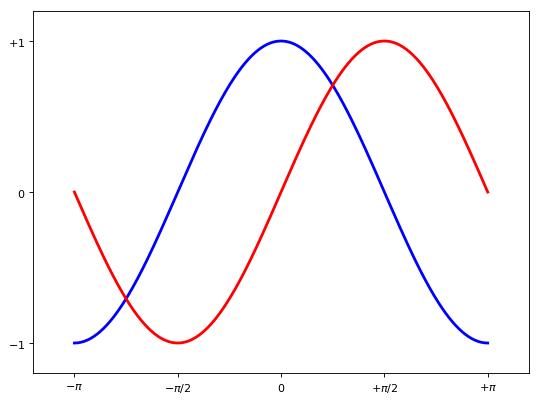移动脊柱 坐标轴线和上面的记号连在一起就形成了脊柱，它记录了数据区域的范围。它们可以放在任意位置，不过至今为止，我们都把它放在图的四边。 实际上每幅图有四条脊柱（上下左右），为了将脊柱放在图的中间，我们必须将其中的两条（上和右）设置为无色，然后调整剩下的两条到合适的位置——数据空间的 0 点。
ax = plt.gca()
ax.spines['right'].set_color('none')
ax.spines['top'].set_color('none')
ax.xaxis.set_ticks_position('bottom')
ax.spines['bottom'].set_position(('data',0))
ax.yaxis.set_ticks_position('left')
ax.spines['left'].set_position(('data',0))

添加图例 我们在图的左上角添加一个图例。为此，我们只需要在 plot 函数里以「键 - 值」的形式增加一个参数。
plt.plot(X, C, color="blue", linewidth=2.5, linestyle="-", label="cosine")
plt.plot(X, S, color="red",  linewidth=2.5, linestyle="-", label="sine")

plt.legend(loc='best')

给一些特殊点做注释 由于给一些点做特殊的注释，代码量多，效果一般，且日常分析较少用，所以这里不再给出具体相关代码，详情看原文。

https://liam.page/2014/09/11/matplotlib-tutorial-zh-cn/

本例完整代码如下（可结合前面注释一起看）：
import numpy as np
import matplotlib.pyplot as plt

plt.figure(figsize=(8,5), dpi=80)
ax = plt.subplot(111)
ax.spines['right'].set_color('none')
ax.spines['top'].set_color('none')
ax.xaxis.set_ticks_position('bottom')
ax.spines['bottom'].set_position(('data',0))
ax.yaxis.set_ticks_position('left')
ax.spines['left'].set_position(('data',0))

X = np.linspace(-np.pi, np.pi, 256,endpoint=True)
C,S = np.cos(X), np.sin(X)

plt.plot(X, C, color="blue", linewidth=2.5, linestyle="-", label="cosine")
plt.plot(X, S, color="red", linewidth=2.5, linestyle="-", label="sine")

plt.xlim(X.min()*1.1, X.max()*1.1)
plt.xticks([-np.pi, -np.pi/2, 0, np.pi/2, np.pi],
[r'$-\pi$', r'$-\pi/2$', r'$0$', r'$+\pi/2$', r'$+\pi$'])

plt.ylim(C.min()*1.1,C.max()*1.1)
plt.yticks([-1, +1],
[r'$-1$', r'$+1$'])

plt.legend(loc='upper left', frameon=False)
plt.savefig('exercice_9.png',dpi=100)
plt.show()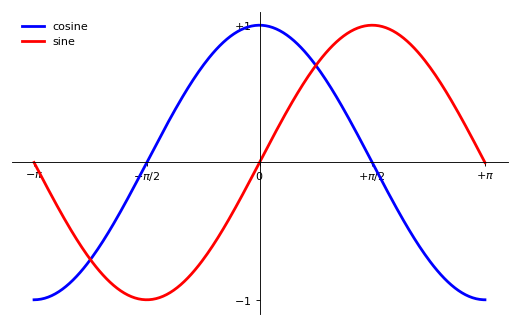图像、子图、坐标轴和记号 到目前为止，我们都用隐式的方法来绘制图像和坐标轴。快速绘图中，这是很方便的。我们也可以显式地控制图像、子图、坐标轴。Matplotlib 中的「图像」指的是用户界面看到的整个窗口内容。在图像里面有所谓「子图」。子图的位置是由坐标网格确定的，而「坐标轴」却不受此限制，可以放在图像的任意位置。我们已经隐式地使用过图像和子图：当我们调用 plot 函数的时候，matplotlib 调用 gca() 函数以及 gcf() 函数来获取当前的坐标轴和图像；如果无法获取图像，则会调用 figure() 函数来创建一个——严格地说，是用 subplot(1,1,1) 创建一个只有一个子图的图像。 图像 所谓「图像」就是 GUI 里以「Figure #」为标题的那些窗口。图像编号从 1 开始，与 MATLAB 的风格一致，而于 Python 从 0 开始编号的风格不同。以下参数是图像的属性：
参数默认值描述num1图像的数量figsizefigure.figsize图像的长和宽（英寸）dpifigure.dpi分辨率（点/英寸）facecolorfigure.facecolor绘图区域的背景颜色dgecolorfigure.edgecolor绘图区域边缘的颜色frameonTrue是否绘制图像边缘
这些默认值可以在源文件中指明。不过除了图像数量这个参数，其余的参数都很少修改。 Matplotlib 也提供了名为 close 的函数来关闭这个窗口。close 函数的具体行为取决于你提供的参数：
不传递参数：关闭当前窗口；传递窗口编号或窗口实例（instance）作为参数：关闭指定的窗口；all：关闭所有窗口。
和其他对象一样，你可以使用 setp 或者是 set_something 这样的方法来设置图像的属性。 子图 你可以用子图来将图样（plot）放在均匀的坐标网格中。用 subplot 函数的时候，你需要指明网格的行列数量，以及你希望将图样放在哪一个网格区域中。此外，gridspec 的功能更强大，你也可以选择它来实现这个功能。
plt.subplot(2,2,1)
plt.xticks([]), plt.yticks([])
plt.text(0.5,0.5, 'subplot(2,2,1)',ha='center',va='center',size=20,alpha=.5)

plt.subplot(2,2,2)
plt.xticks([]), plt.yticks([])
plt.text(0.5,0.5, 'subplot(2,2,2)',ha='center',va='center',size=20,alpha=.5)

plt.subplot(2,2,3)
plt.xticks([]), plt.yticks([])
plt.text(0.5,0.5, 'subplot(2,2,3)',ha='center',va='center',size=20,alpha=.5)

plt.subplot(2,2,4)
plt.xticks([]), plt.yticks([])
plt.text(0.5,0.5, 'subplot(2,2,4)',ha='center',va='center',size=20,alpha=.5)

plt.show()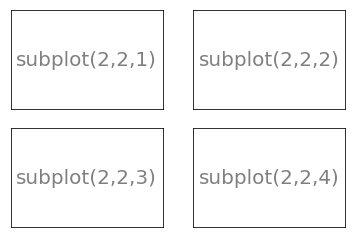坐标轴 坐标轴和子图功能类似，不过它可以放在图像的任意位置。因此，如果你希望在一副图中绘制一个小图，就可以用这个功能。
plt.axes([0.1,0.1,.8,.8])
plt.xticks([]), plt.yticks([])
plt.text(0.6,0.6, 'axes([0.1,0.1,.8,.8])',ha='center',va='center',size=20,alpha=.5)

plt.axes([0.2,0.2,.3,.3])
plt.xticks([]), plt.yticks([])
plt.text(0.5,0.5, 'axes([0.2,0.2,.3,.3])',ha='center',va='center',size=16,alpha=.5)

plt.show()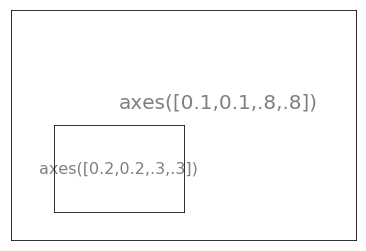plt.axes([0.1,0.1,.5,.5])
plt.xticks([]), plt.yticks([])
plt.text(0.1,0.1, 'axes([0.1,0.1,.8,.8])',ha='left',va='center',size=16,alpha=.5)

plt.axes([0.2,0.2,.5,.5])
plt.xticks([]), plt.yticks([])
plt.text(0.1,0.1, 'axes([0.2,0.2,.5,.5])',ha='left',va='center',size=16,alpha=.5)

plt.axes([0.3,0.3,.5,.5])
plt.xticks([]), plt.yticks([])
plt.text(0.1,0.1, 'axes([0.3,0.3,.5,.5])',ha='left',va='center',size=16,alpha=.5)

plt.axes([0.4,0.4,.5,.5])
plt.xticks([]), plt.yticks([])
plt.text(0.1,0.1, 'axes([0.4,0.4,.5,.5])',ha='left',va='center',size=16,alpha=.5)

plt.show()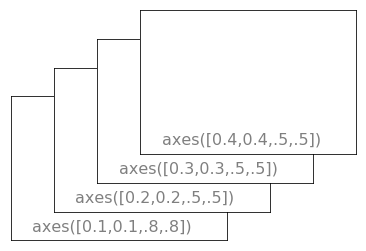记号 良好的记号是图像的重要组成部分。Matplotlib 里的记号系统里的各个细节都是可以由用户个性化配置的。你可以用 Tick Locators 来指定在那些位置放置记号，用 Tick Formatters 来调整记号的样式。主要和次要的记号可以以不同的方式呈现。默认情况下，每一个次要的记号都是隐藏的，也就是说，默认情况下的次要记号列表是空的——NullLocator。
后记 当然Matplotlib 不仅仅只有这些操作，它可以绘制许许多多的图，同时，Python中绘图的工具也不限于Matplotlib。想要学习更多内容，官网的教程是一个不错的选择。以下推荐两个官网教程： matplotlib（https://matplotlib.org/） seaborn（http://seaborn.pydata.org/）
展开全文Python
• 1.找到或新建你的项目文件夹 2.将下载好的.whl文件复制到你的项目文件夹 3.打开命令窗口，切换到该项目文件夹，之后使用pip命令安装matplotlib
       作为一个新手小白，将要学习编写数据可视化项目中，刚开始就出现棘手的问题，关于安装matplotlib。在我搜集大量资料后总结安装matplotlib过程并分享供大家参考。
数据科学家使用Python编写了一系列令人印象深刻的可视化和分析工具，其中很多也可供学习使用。最流行的工具之一是matplotlib，它是一个数学会图库，我们将使用它来制作简单的图表，比如折线图和散点图等。
准备工作：
1.安装python2.下载Visual Studio3.下载对于Python版本的matplotlib（.whl）安装文件
安装步骤：
1.查看Python版本2.安装wheel工具3.安装Visual Studio（Windows）
安装方法：
1.找到或新建你的项目文件夹2.将下载好的.whl文件复制到你的项目文件夹3.打开命令窗口，切换到该项目文件夹，之后使用pip命令安装matplotlib
安装命令：
1：pip install 文件名 （或者）2：python -m pip install --user matplotlib(.whl)安装文件
详细安装说明：
先查看电脑有没有安装wheel，在命令行输入wheel，无相关信息表示没安装。需要先安装wheel 在命令行输入pip install wheel。若提示pip版本号过低。看下面蓝色图片，进行升级更新最新pip。然后再来安装wheel，如下图：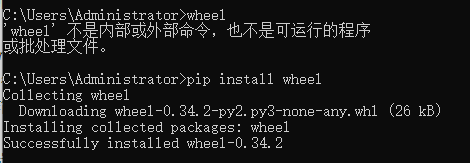安装wheel过程中可能出现的错误：
无法正确安装wheel，说明pip版本过低 若pip版本过低，包括wheel、matplotlib在内的很多包否无法安装。 解决办法：在命令行输入 python -m pip install --upgrade pip来升级pip版本。 看到Successfully字样即升级成功，如下图：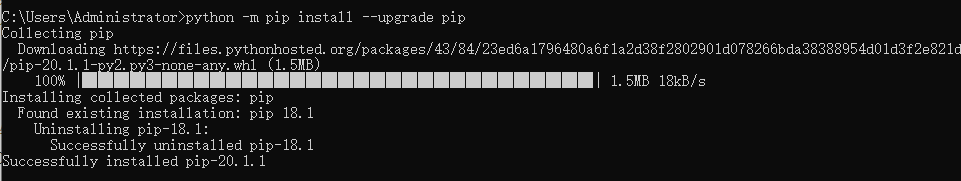升级成功后可以正常的用pip安装wheel。包括用pip安装matplotlib包也需要升级pip版本。

前期工作做完后开始正式安装matplotlib包：
1.查看python版本，即，在命令行输入Python，查看Python版本，如下图。根据版本去matplotlib官网下载相应的matplotlib文件。例如我的是Python3.7（Windows版本），则需要下载matplotlib-3.2.2-cp37-cp37m-win_amd64.whl。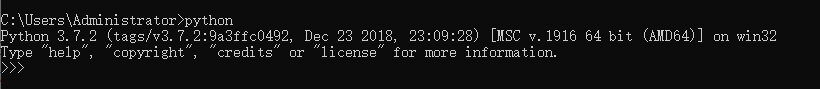2.将这个.whl文件复制到你的项目文件夹中。打开命令窗口，并切换到该项目文件夹。  >cd 此处是你的项目文件夹地址
例如，我的项目文件夹是PyCode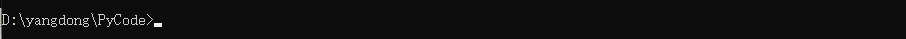PS：关于命令行如何进入项目文件夹，我再下一篇博客会详细说明。
3.在命令行进入项目文件夹之后使用pip来安装matplotlib： 项目文件夹> python -m pip install --user matplotlib-3.2.2-cp37-cp37m-win_amd64.whl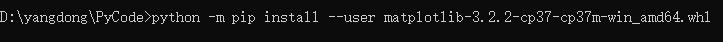matplotlib开始安装，如下图：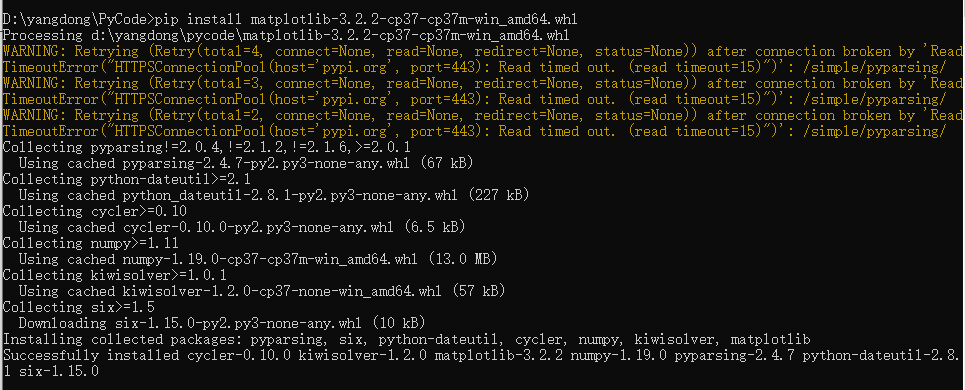出现Successfully 表示安装成功。

matplotlib安装过程中我出现几次错误:
如（1）：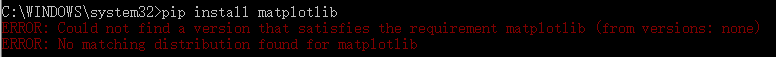我的原因是：下载相关文件时连接中断，网络超时导致。 解决：多安装几遍就好了。
错误（2）：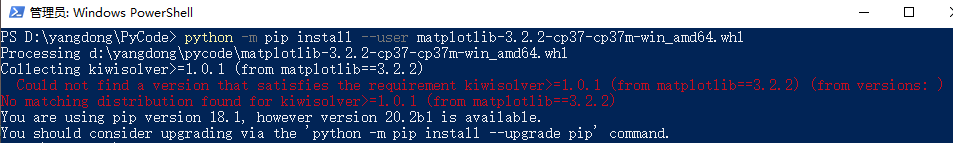原因之一：pip版本过低。 解决：参考上面升级pip版本。

安装成功后测试：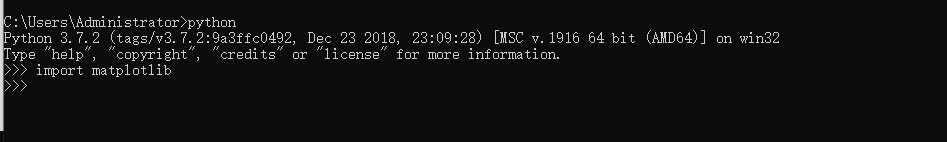首先使用命令python启动一个终端会话，再尝试导入matplotlib。如果没有出现任何错误消息，就说明系统安装了matplotlib。 PS：我并未下载安装Visual Studio,但是教科书上说是需要安装，不知道为什么，可能需要其中的环境(瞎猜的)。最好是根据我开头列的准备工作来。

安装Anaconda
使用pip安装时难免出现各种问题，同样也可以下载Anaconda安装器，Anaconda中带有matplotlib包。按照Anaconda官网下载页上的说明进行安装。        安装完成后，验证所有设置是否都正确。进入命令行，输入python来启动Python解释器，应该可以看到符合你下载的Anaconda版本信息。
安装及更新Python包
Anaconda中带有matplotlib包，对于其他的Anaconda并不包含的额外Python包。通过命令行进行安装：
conde install *package_name*

如果不奏效你可以使用pip包管理工具进行安装：
pip install *package_name*

你还可以使用conda update 命令来更新包：
conda update *package_name*

pip install --upgrade *package_name*

当使用Anaconda或者Miniconda时，最好还是使用conda进行更新。

参考资料：

《Python编程-从入门到实践》 《利用Python进行数据分析》   B站专业技术up主

展开全文python 人工智能 可视化
• TutorialsPoint Matplotlib 教程.epub互联网
• 关于Python-Matplotlib绘图的教程，包括中文英文两个版本，英文版本可以在 https://matplotlib.org/Matplotlib.pdf 参考，中文版本来自GitHub，资源网址 https://github.com/jdk6979/matplotlib-intro-tut-zh 。
• 这是一个matplotlib学习教程 里面的代码是关于matplotlib的Python绘图学习基础 里面的案例都是可以直接执行
• matplotlib是基于Python的绘图库，广泛用于Python科学计算界。它完整支持二维绘图以及部分支持三维绘图。该绘图库致力于能适应广泛的用户需求。它可以根据所选的用户接口工具来嵌入绘图算法。与此同时，对于使用GTK+...
matplotlib是基于Python的绘图库，广泛用于Python科学计算界。它完整支持二维绘图以及部分支持三维绘图。该绘图库致力于能适应广泛的用户需求。它可以根据所选的用户接口工具来嵌入绘图算法。与此同时，对于使用GTK+、Qt、Tk、FLTK、wxWidgets与Cocoa的所有主要桌面操作系统，matplotlib能支持交互式绘图。在Python的交互式shell中，我们可以使用简单的、过程式的命令交互式地调用matplotlib来生成图形，与使用Mathematica、IDL或者MATLAB绘图非常相似。matplotlib也可以嵌入到无报文头的Web服务器中，以提供基于光栅（如PNG格式）与向量（如Postscript、PDF以及纸面效果很好的SVG格式）这两种格式的图形硬拷贝。
一、历史溯源—— 硬件锁问题
我们其中一位开发者（John Hunter）与他的研究癫痫症的同事们试图在不借助专有软件的情况下进行脑皮层电图（ECoG）分析，于是便有了最初的matplotlib。John Hunter当时所在的实验室只有一份电图分析软件的许可证，但有各式各样的工作人员，如研究生、医科学生、博士后、实习生、以及研究员，他们轮流共享该专有软件的硬件电子锁。生物医学界广泛使用MATLAB进行数据分析与可视化，所以Hunter着手使用基于MATLAB的matplotlib来代替专有软件，这样很多研究员都可以使用并且对其进行扩展。但是MATLAB天生将数据当作浮点数的数组来处理。然而在实际情况中，癫痫手术患者的医疗记录具有多种数据形式（CT、MRI、ECoG与EEG等），并且存储在不同的服务器上。MATLAB作为数据管理系统勉强能应付这样的复杂性。由于感到MATLAB不适合于这项任务，Hunter开始编写一个新的建立在用户接口工具GTK+（当时是Linux下的主流桌面视窗系统）之上的Python应用程序。
所以matplotlib这一GTK+应用程序最初便被开发成EEG/ECoG可视化工具。这样的用例决定了它最初的软件架构。matplotlib最初的设计也服务于另一个目的：代替命令驱动的交互式图形生成（这一点MATLAB做得很好）工具。MATLAB的设计方法使得加载数据文件与绘图这样的任务非常简单，而要使用完全面向对象的API则会在语法上过于繁琐。所以matplotlib也提供状态化的脚本编程接口来快速、简单地生成与MATLAB类似的图形。因为matplotlib是Python库，所以用户可以使用Python中各种丰富的数据结构，如列表、辞典与集合等等。二、 matplotlib软件架构概述
顶层的matplotlib对象名为Figure，它包含与管理某个图形的所有元素。matplotlib必须完成的一个核心架构性任务是实现Figure的绘制与操作框架，并且做到该框架与Figure到用户视窗接口或硬拷贝渲染行为是分离的。这使得我们可以为Figure添加越来越复杂的特性与逻辑，同时保持“后端”或输出设备的相对简化。matplotlib不仅封装了用于向多种设备渲染的绘图接口，还封装了基本事件处理以及多数流行的用户界面工具的视窗功能。因此，用户可以创建相当丰富的交互式图形算法与用户界面工具（用到可能存在的鼠标与键盘），而又不必修改matplotlib已经支持的6种界面工具。
要实现这些，matplotlib的架构被逻辑性地分为三层。这三层逻辑可以视为一个栈。每层逻辑知道如何与其下的一层逻辑进行通信，但在下层逻辑看来，上层是透明的。这三层从底向上分别为：后端、美工与脚本。

1、 后端
matplotlib逻辑栈最底层是后端，它具体实现了下面的抽象接口类：
FigureCanvas对绘图表面（如“绘图纸”）的概念进行封装。Renderer执行绘图动作（如“画笔”）。Event处理键盘与鼠标事件这样的用户输入。
对于如Qt这样的用户界面工具，FigureCanvas中包含的具体实现可以完成三个任务：将自身嵌入到原生的Qt视窗（QtGui.QMainWindow）中，能将matplotlib的Renderer命令转换到canvas上（QtGui.QPainter），以及将原生Qt事件转换到matplotlib的Event框架下（后者产生回调信号让上行监听者进行处理）。抽象基类定义在matplotlib.backend_bases中，且所有派生类都定义在如matplotlib.backends.backend_qt4agg这样的专用模块中。对于专门生成硬拷贝输出（如PDF、PNG、SVG或PS）的纯图像后端而言，FigureCanvas的实现可能只是简单地建立一个类似文件的对象，其中定义默认的文件头、字体与宏函数，以及Renderer创建的个别对象（如直线、文本与矩形等）。
Renderer的任务是提供底层的绘图接口，即在画布上绘图的动作。上文已经提到，最初的matplotlib程序是一个基于GTK+的ECoG查看器，而且很多早期设计灵感都源自当时已有的GDK/GTK+的API。最初Renderer的API源自GDK的Drawable接口，后者实现了draw_point、draw_line、draw_rectangle、draw_image、draw_polygon以及draw_glyphs这样的基本方法。我们完成的每个不同后端——最早有PostScript与GD——都实现了GDK的Drawable，并将其转换为独立于后端的原生绘图命令。如上所述，这毫无必要地增加了后端的实现复杂度，原因是单独实现Drawable造成函数泛滥。此后，Renderer已经被极大的简化，将matplotlib移植到新的用户界面或文件格式已经是非常简单的过程。
一个对matplotlib有利的设计决定是支持使用C++模板库Anti-Grain Geometry（缩写为agg[She06]）的基于像素点的核心渲染器。这是一个高性能库，可以进行2D反锯齿渲染，生成的图像非常漂亮。matplotlib支持将agg后端渲染的像素缓存插入到每种支持的用户界面中，所以在不同的UI与操作系统下都能得到精确像素点的图形。因为matplotlib生成的PNG输出也使用agg渲染器，所以硬拷贝与屏幕显示完全相同，也就是说在不同的UI与操作系统下，PNG的输出所见即所得。
matplotlib的Event框架将key-press-event或mouse-motion-event这样的潜在UI事件映射到KeyEvent或MouseEvent类。用户可以连接到这些事件进行函数回调，以及图形与数据的交互，如要pick一个或一组数据点，或对图形或其元素的某方面性质进行操作。下面的示例代码演示了当用户键入‘t’时，对Axes窗口中的线段进行显示开关。
import numpy as np
import matplotlib.pyplot as plt

def on_press(event):
if event.inaxes is None: return
for line in event.inaxes.lines:
if event.key=='t':
visible = line.get_visible()
line.set_visible(not visible)
event.inaxes.figure.canvas.draw()

fig, ax = plt.subplots(1)

fig.canvas.mpl_connect('key_press_event', on_press)

ax.plot(np.random.rand(2, 20))

plt.show()

对底层UI事件框架的抽象使得matplotlib的开发者与最终用户都可以编写UI事件处理代码，而且“一次编写，随处运行”。譬如，在所有用户界面下都可以对matplotlib图像进行交互式平移与放缩，这种交互式操作就是在matplotlib的事件框架下实现的。
2 、Artis层
（称之为“ 美工”其实是一个汉化的形象说法，因为“ 在哪里画，用什么画，画什么图？ ”这不正是美工的工作么？）
Artist层次结构处于matplotlib的中间层，负责很大一部分繁重的计算任务。延续之前将后端的FigureCanvas看作画纸的比喻，Artis对象知道如何用Renderer（画笔）在画布上画出墨迹。matplotlib中的Figure就是一个Artist对象实例。标题、直线、刻度标记以及图像等等都对应某个Artist实例（如图11.3）。Artist的基类是matplotlib.artist.Artist，其中包含所有Artist的共享属性，包括从美工坐标系统到画布坐标系统的变换（后面将详细介绍）、可见性、定义用户可绘制区域的剪切板、标签，以及处理“选中”这样的用户交互动作的接口，即在美工层检测鼠标点击事件。Artist层于后端之间的耦合性存在于draw方法中。譬如，下面假想的SomeArtist类是Artist的子类，它要实现的关键方法是draw，用来传递给后端的渲染器。Artist不知道渲染器要向哪种后端进行绘制（PDF、SVG与GTK+绘图区等），但知道Renderer的API，并且会调用适当的方法（draw_text或draw_path）。因为Renderer能访问画布，并且知道如何绘制，所以draw方法将Artist的抽象表示转换为像素缓存中的颜色、SVG文件中的轨迹或者其他具体表示。
class SomeArtist(Artist):
'An example Artist that implements the draw method'

def draw(self, renderer):
"""Call the appropriate renderer methods to paint self onto canvas"""
if not self.get_visible():  return

# create some objects and use renderer to draw self here
renderer.draw_path(graphics_context, path, transform)

该层次结构中有两种类型的Artist。基本Artist表示我们在图形中能看到的一类对象，如Line2D、Rectangle、Circle与Text。复合Artist是Artist的集合，如Axis、Tick、Axes与Figure。每个复合Artsit可能包含其他复合Artist与基本Artist。譬如，Figure包含一个或多个Axes，并且Figure的背景是基本的Rectangle。
最重要的复合Artist是Axes，其中定义了大多数matplot的绘图方法。Axes不仅仅包含大多数构成绘图背景（如标记、轴线、网格线、色块等）的图形元素，还包括了大量生成基本Artist并添加到Axes实例中的帮助函数。譬如，表11.1列出了一些Axes函数，这些函数进行对象的绘制，并将它们存储在Axes实例中。
表11.1：Axes的方法样例及其创建的Artist实例
| 方法 | 创建对象 | 存储位置 | | Axes.imshow | 一到多个matplotlib.image.AxesImage | Axes.images | | Axes.hist | 大量matplotlib.patch.Rectangle | Axes.patches | | Axes.plot | 一到多个matplotlib.lines.Line2D | Axes.lines |
下面这个简单的Python脚本解释了以上架构。它定义了后端，将Figure链接至该后端，然后使用数组库numpy创建10,000个正太分布的随机数，最后绘制出它们的柱状图。
# Import the FigureCanvas from the backend of your choice
#  and attach the Figure artist to it.
from matplotlib.backends.backend_agg import FigureCanvasAgg as FigureCanvas
from matplotlib.figure import Figure
fig = Figure()
canvas = FigureCanvas(fig)

# Import the numpy library to generate the random numbers.
import numpy as np
x = np.random.randn(10000)

# Now use a figure method to create an Axes artist; the Axes artist is
#  added automatically to the figure container fig.axes.
# Here "111" is from the MATLAB convention: create a grid with 1 row and 1
#  column, and use the first cell in that grid for the location of the new
#  Axes.

# Call the Axes method hist to generate the histogram; hist creates a
#  sequence of Rectangle artists for each histogram bar and adds them
#  to the Axes container.  Here "100" means create 100 bins.
ax.hist(x, 100)

# Decorate the figure with a title and save it.
ax.set_title('Normal distribution with $\mu=0, \sigma=1$')
fig.savefig('matplotlib_histogram.png')

3 、脚本层（pyplot）
使用以上API的脚本效果很好，尤其是对于程序员而言，并且在编写Web应用服务器、UI应用程序或者是与其他开发人员共享的脚本时，这通常是比较合适的编程范式。对于日常用途，尤其对于非专业程序员而要完成一些交互式的研究工作的实验科学家而言，以上API的语法可能有些难以掌握。大多数用于数据分析与可视化的专用语言都会提供轻量级的脚本接口来简化一些常见任务。matplotlib在其matplotlib.pyplot接口中便实现了这一点。以上代码改用pyplot之后如下所示。
import matplotlib.pyplot as plt
import numpy as np

x = np.random.randn(10000)
plt.hist(x, 100)
plt.title(r'Normal distribution with $\mu=0, \sigma=1$')
plt.savefig('matplotlib_histogram.png')
plt.show()图11.4：用pyplot绘制的柱状图
pyplot是一个状态化接口，大部分工作是处理样本文件的图形与坐标的生成，以及与所选后端的连接。它还维护了模块级的内部数据结构。这些数据结构表示了直接接收绘图命令的当前图形与坐标
下面仔细分析示例脚本中比较重要的几行，观察其内部状态的管理方式。
import matplotlib.pyplot as plt：当pyplot模块被加载时，它分析本地配置文件。配置文件除了完成一些其他工作外，主要声明了默认的后端。可能是类似QtAgg的用户接口后端，于是上面的脚本将导入GUI框架并启动嵌入了图形的Qt窗口；或者可以是一个类似Agg的纯图像后端，这样脚本会生成硬拷贝输出然后退出。plt.hist(x, 100)：这是脚本中第一个绘图命令。pyplot会检测其内部数据结构已查看是否存在当前Figure实例。如果存在，则提取当前Axes，并将绘图行为导向Axes.hist的API调用。在该脚本中不存在Figure实例，所以会生成一个FIgure与Axes，并将它们设为当前值，然后将绘图行为导向Axes.hist。 plt.hist(x, 100): This is the first plotting command in the script. pyplot will check its internal data structures to see if there is a current Figure instance. If so, it will extract the current Axes and direct plotting to the Axes.hist API call. In this case there is none, so it will create a Figure and Axes, set these as current, and direct the plotting to Axes.hist. plt.title(r'Normal distribution with $\mu=0, \sigma=1$'): As above, pyplot will look to see if there is a current Figure and Axes. Finding that there is, it will not create new instances but will direct the call to the existing Axes instance method Axes.set_title. plt.show(): This will force the Figure to render, and if the user has indicated a default GUI backend in their configuration file, will start the GUI mainloop and raise any figures created to the screen.
三、总结
1、matplotlib是一个非常庞大的系统，而且从诞生到现在繁荣发展经历了几十年的时间，里面所涉及到的软件架构很是复杂。
2、matplotlib的使用可以从三个层面上去入手，即“后端——美工——脚本”。
（1）后端：我们当然可以从最底层的后端搭建一个图形，负责软件与绘图硬件的交互，图形的各种渲染等等，这异常困难，而且对于程序员的开发效率来说，十分缓慢，因为绘图的框架整个都要自己去搭建，很麻烦。
（2）美工：这是在后端的基础之上的进一步封装，有一点类似于GDI+绘图方式，我们只关心“ 在哪里画 、用什么笔、 画什么图 ”，所用的笔，所用的颜料，画的图形类型（折线图，条形图，散点图等等）这些已经封装好了，封装是通过一系列的类去完成的。
（3）脚本：脚本是matplotlib的更高层封装，也就是我们平时所用的pyplot模块的高级封装，使用脚本，我们再也不用关心这个图形到底是怎么画的了，我们只需要关心画图的效果即可。适用“ 后端 ”、“ 美工 ”、要很多代码才能完成的事，使用脚本一句话就可以实现，如：
plt.plot(x,y,color='red',linewidth=3,linestyle='--')
就是这么简单的一句话如果要自己从头搭建，是一件很困难的事情。
展开全文• 背景： 使用pip install SciPy的格式安装python函数库SciPy的时候，发现老是报错，从网上找信息也没找到合适的解决办法，最后使用whl格式文件安装成功。 过程： 本人事先电脑安装了python3.5.2，电脑是64位的。...
• AnatomyOfMatplotlib, 针对SciPy会议开发的Matplotlib教程剖析 简介本教程是关于如何教用户matplotlib库的完整设想。 希望本教程可以作为未来matplotlib文档重构的启示。 另外，我还有一些关于如何改进本教程的想法...
• 进入正文一、概述 matplotlib是Python生态圈里面最为流行的图形可视化库，提供了庞大而且完善的绘图功能，在绝大多数情况下，基本上只要是你想要实现的功能，...
• matplotlib-tutorial:Corey Schafer的matplotlib教程中的代码Python
• 系列文章目录 【matplotlib教程】简介、安装、示例 【matplotlib教程】绘图样式，文本线型、轴刻度 【matplotlib教程】使用各种类型数据绘图 【matploblib教程】一文带你码遍各种类型三维图 【matplotlib教程】图像...python 数据分析 数据可视化
• 要设置本教程的环境，请使用 $conda env create -f environment.yml 或者$ conda create -n mpl-tutorial -c anaconda matplotlib pandas pytables h5py ipython scipy python=3.6 对于* nix \$ source ...Python
• 画图python库ｍａｔｐｌｏｔｌｉｂ教程,里面包含wx，pyqt，和网络使用等
• 主要介绍了Python Matplotlib简易教程(小白教程)，文中通过示例代码介绍的非常详细，对大家的学习或者工作具有一定的参考学习价值，需要的朋友们下面随着小编来一起学习学习吧
• 50种常用的matplotlib可视化，再也不用担心模型背着我乱跑了 https://blog.csdn.net/sophia_11/article/details/86326172 ...
• 一直将matplotlib当做一个工具来用，因为没有了解到它的特性，所以一直学得不系统，导致用到的时候经常要查官方文档。这里翻译一个官方推荐的matplotlib的介绍文档。 猛戳进入原文链接 文档中包含的内容： 简介 ...python...